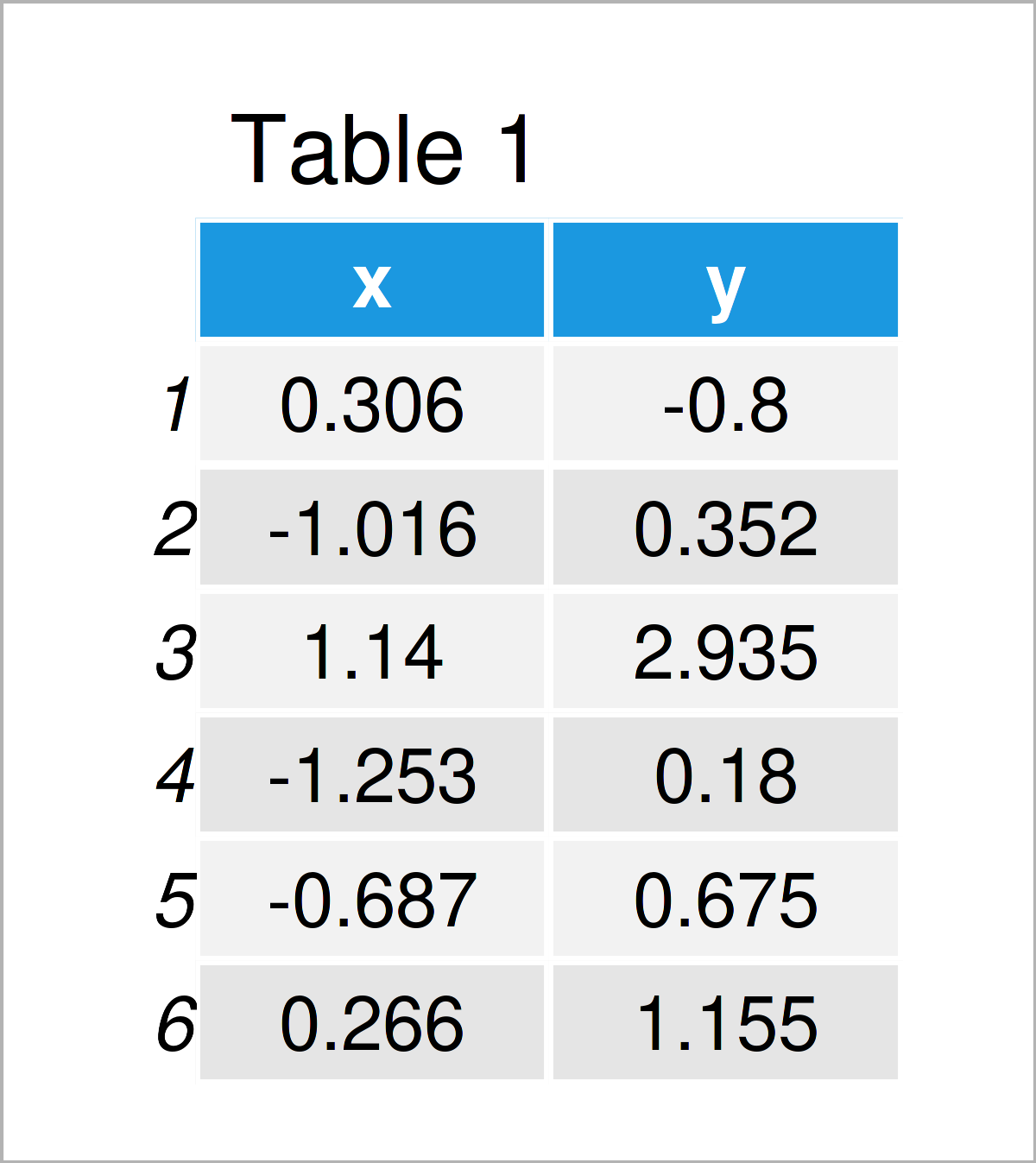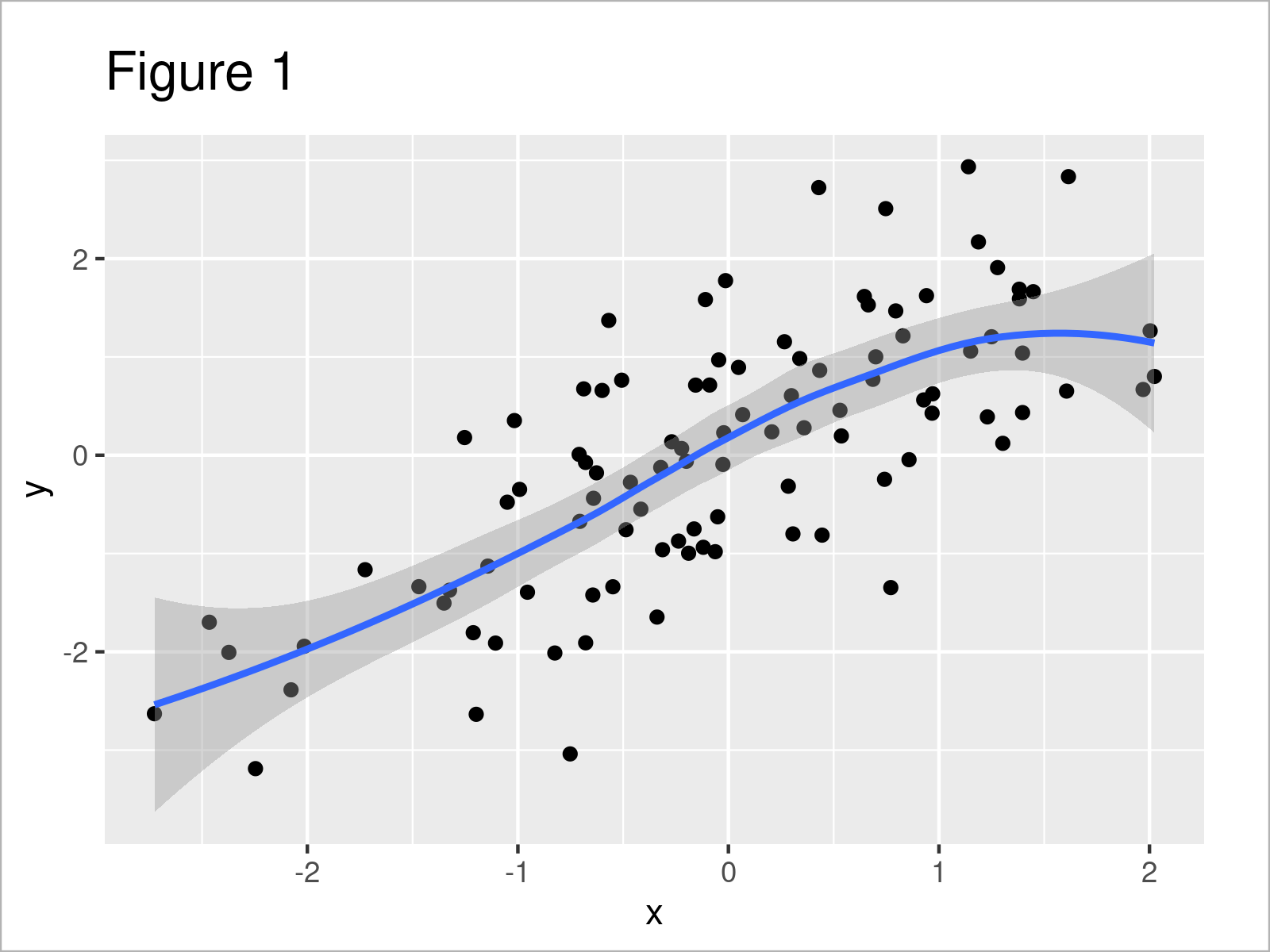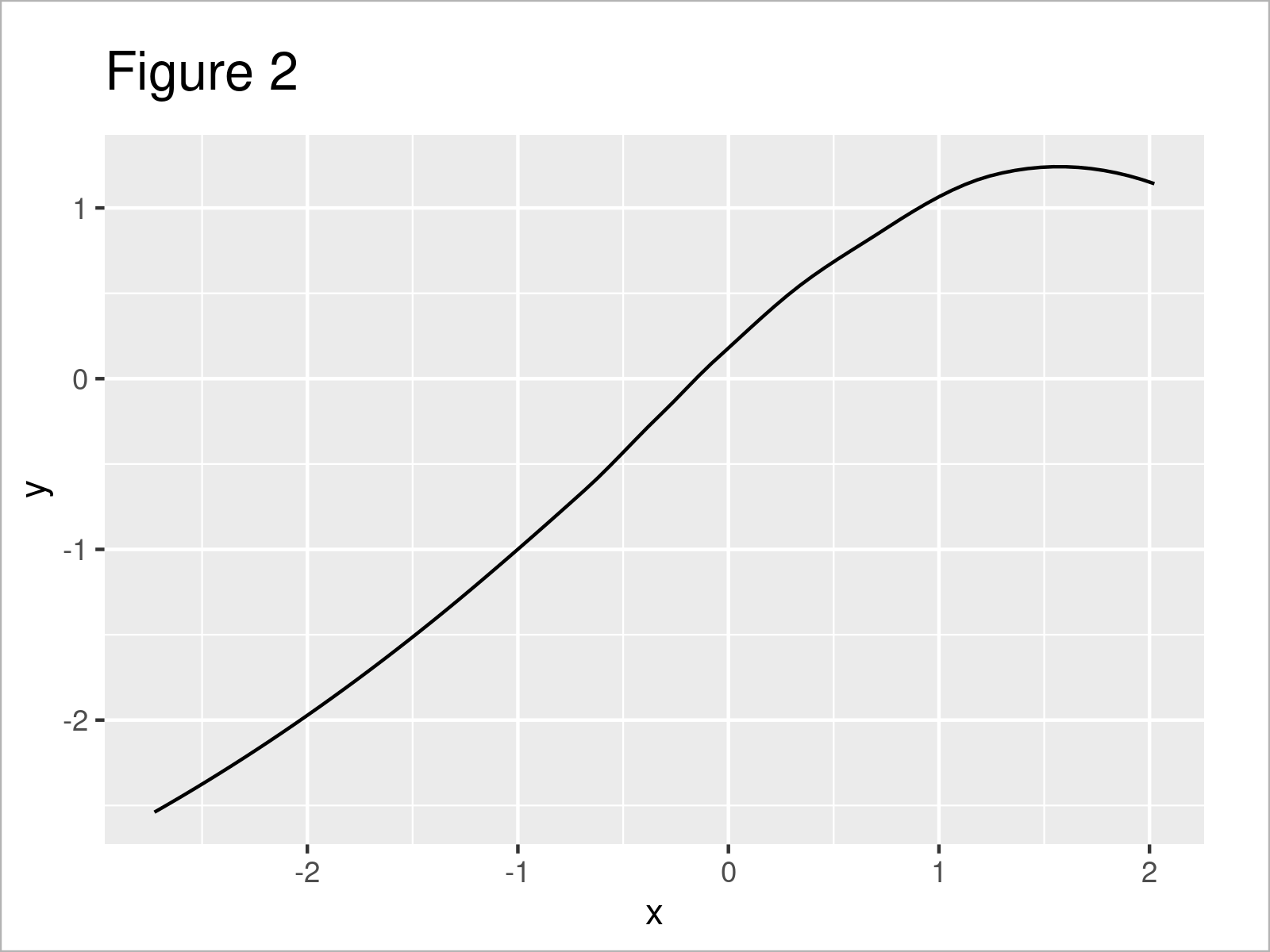# Extract stat_smooth Regression Line Fit from ggplot2 Plot in R (Example)

In this article, I’ll explain how to get the line fit coordinates of a ggplot2 plot in the R programming language.

The tutorial consists of this content:

## Example Data, Packages & Default Graph

The first step is to create some data that we can use in the examples below:

```set.seed(23876382)                          # Create example data
x <- rnorm(100)
y <- x + rnorm(100)
data <- data.frame(x, y)Table 1 illustrates that our example data has two numerical columns called “x” and “y”.

In order to use the functions of the ggplot2 package, we also need to install and load ggplot2:

```install.packages("ggplot2")                 # Install & load ggplot2 package
library("ggplot2")```

Next, we can create a graphic of the data:

```ggp <- ggplot(data, aes(x, y)) +            # Draw ggplot2 plot with stat_smooth line
geom_point() +
stat_smooth()
ggp```As shown in Figure 1, the previous R syntax has plotted a ggplot2 scatterplot with a line created by the stat_smooth function.

Note: In this tutorial, we have used the default specification of the stat_smooth function (i.e. method = ‘loess’ and formula ‘y ~ x’). However, the following R code could also be applied in case we would have used another method such as linear regression model (method = “lm”) or a generalized linear model (method = “glm”). Furthermore, we could also use the geom_smooth function instead of stat_smooth.

However, let’s move on to the example code…

## Example: Extract stat_smooth Regression Line Fit from ggplot2 Plot Using ggplot_build() Function

This example shows how to get the x- and y-coordinates of a stat_smooth line in a ggplot2 plot.

For this task, we can apply the ggplot_build function as shown below:

```ggp_data <- ggplot_build(ggp)\$data[]     # Extract information about plot
#           x         y      ymin      ymax        se flipped_aes PANEL group  colour   fill size linetype weight alpha
# 1 -2.726269 -2.539296 -3.631610 -1.446982 0.5501666       FALSE     1    -1 #3366FF grey60    1        1      1   0.4
# 2 -2.666148 -2.496717 -3.515914 -1.477519 0.5133401       FALSE     1    -1 #3366FF grey60    1        1      1   0.4
# 3 -2.606028 -2.453355 -3.403513 -1.503196 0.4785674       FALSE     1    -1 #3366FF grey60    1        1      1   0.4
# 4 -2.545908 -2.409207 -3.294451 -1.523964 0.4458714       FALSE     1    -1 #3366FF grey60    1        1      1   0.4
# 5 -2.485787 -2.364273 -3.188773 -1.539772 0.4152770       FALSE     1    -1 #3366FF grey60    1        1      1   0.4
# 6 -2.425667 -2.318549 -3.086530 -1.550568 0.3868098       FALSE     1    -1 #3366FF grey60    1        1      1   0.4```

As you can see based on the previous RStudio console output, the ggplot_build function has created a data set containing different information about our plot.

In our case, we are interested in the x and y columns of this output, since those two columns contain the x- and y-coordinates of our stat_smooth line.

We can double-check that by drawing the values in the variables x and y in a line plot:

```ggp_fit <- ggplot(ggp_data, aes(x, y)) +    # Redraw stat_smooth line
geom_line()
ggp_fit```By executing the previous R programming syntax we have created Figure 2, i.e. a ggplot2 line plot showing only the stat_smooth line of our original plot.

Note that the axis limits of Figure 2 are different compared to Figure 1 – That’s why the stat_smooth line may look a little different.

## Video & Further Resources

If you need further info on the examples of this page, you might watch the following video on the Statistics Globe YouTube channel. I’m explaining the R programming codes of this tutorial in the video.

Please accept YouTube cookies to play this video. By accepting you will be accessing content from YouTube, a service provided by an external third party.If you accept this notice, your choice will be saved and the page will refresh.

In addition, you may read the related articles on this website. I have published numerous other articles already.

In this tutorial you have learned how to extract the fitted values shown in a regression line in R programming. In case you have further questions, tell me about it in the comments section.

Subscribe to the Statistics Globe Newsletter